Search by Topic

Resources tagged with Representing similar to Different Sizes:

Filter by: Content type:
Age range:
Challenge level:

There are 15 resultsDifferent Sizes

Age 5 to 11 Challenge Level:

A simple visual exploration into halving and doubling.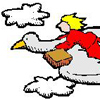A Flying Holiday

Age 7 to 11 Short Challenge Level:

Follow the journey taken by this bird and let us know for how long and in what direction it must fly to return to its starting point.Catrina's Cards

Age 5 to 7 Challenge Level:

How would you find out how many football cards Catrina has collected?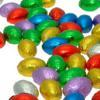Let Us Divide!

Age 7 to 11 Challenge Level:

Look at different ways of dividing things. What do they mean? How might you show them in a picture, with things, with numbers and symbols?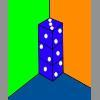Dice in a Corner

Age 7 to 11 Challenge Level:

How could you arrange at least two dice in a stack so that the total of the visible spots is 18?Birthday Sharing

Age 5 to 7 Challenge Level:

It's Sahila's birthday and she is having a party. How could you answer these questions using a picture, with things, with numbers or symbols?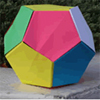Dodecamagic

Age 7 to 11 Challenge Level:

Here you see the front and back views of a dodecahedron. Each vertex has been numbered so that the numbers around each pentagonal face add up to 65. Can you find all the missing numbers?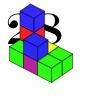28 and It's Upward and Onward

Age 7 to 11 Challenge Level:

Can you find ways of joining cubes together so that 28 faces are visible?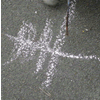Children's Mathematical Graphics: Understanding the Key Concept

Age 5 to 7

In this article for teachers, Elizabeth Carruthers and Maulfry Worthington explore the differences between 'recording mathematics' and 'representing mathematical thinking'.3D Stacks

Age 7 to 14 Challenge Level:

Can you find a way of representing these arrangements of balls?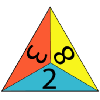One Big Triangle

Age 5 to 7 Challenge Level:

Make one big triangle so the numbers that touch on the small triangles add to 10.Going for Gold

Age 7 to 11 Challenge Level:

Looking at the 2012 Olympic Medal table, can you see how the data is organised? Could the results be presented differently to give another nation the top place?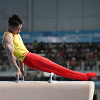Olympic Turns

Age 7 to 11 Challenge Level:

This task looks at the different turns involved in different Olympic sports as a way of exploring the mathematics of turns and angles.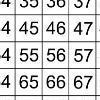That Number Square!

Age 5 to 11 Challenge Level:

Exploring the structure of a number square: how quickly can you put the number tiles in the right place on the grid?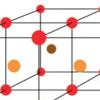Finding 3D Stacks

Age 7 to 11 Challenge Level:

Can you find a way of counting the spheres in these arrangements?